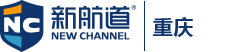4001859090

## 备考小课堂-GMAT数学核心知识点之“数”的基本性质

2020-09-01 15:09 来源：重庆编辑

1整数及相关性质(integers)

2分数、小数以及百分比(fractions， decimals， percent)

3集合以及相关问题(problems involving sets)

(一)数的性质之“因数”

1How many different prime numbers are factors of the positive integer n?

(1) Four different prime numbers are factors of 2n.

(2) Four different prime numbers are factors of n 2.

A Statement(1) ALONE is sufficient， but statement(2) alone is not sufficient.

B Statement(2) ALONE is sufficient， but statement(1) alone is not sufficient.

C BOTH statement TOGETHER are sufficient， but NEITHER

statement ALONE is sufficient.

D EACH statement ALONE is sufficient.

F Statements(1) and(2) TOGETHER are NOT sufficient.

【题目翻译】

1)2n有4个不同的质因数

2)n2有4个不同的质因数

【题目分析】

1)2n有4个质因子，比如2*3*5*7=210，210有4个质因子，即2n有4个质因子，此时n=105有3个质因子，分别为3*5*7，结论为n比2n少一个质因子。然而，当2n=2*2*3*5*7=420的时候，n=210，结论为2n和n的质因子数目一样。所以关键在于2n包含几个2.

2)n^2有4个质因子，比如2*2*3*3*5*5*7*7=44100，那么

n=2*3*5*7=210结论是n^2和n的质因子一定相同，数目质因子数目一定相同。

【错误分析】

【知识点】

2是最小的质数(prime number) ； n^2跟n本身拥有相同多的质因数(prime factor)。

(二)数的性质之“余数”

Ifn and mare positive integers， what is the remainder when

3^(4n+2+m) is divided by 10?

(1)n=2

(2)m=1

A Statement(1) ALONE is sufficient， but statement(2) alone is

not sufficient.

B Statement(2) ALONE is sufficient， but statement(1) alone is

not sufficient.

C BOTH statement TOGETHER are sufficient， but NEITHER

statement ALONE is sufficient.

D EACH statement ALONE is sufficient.

E Statements(1) and(2) TOGETHER are NOT suff cient.

【题目翻译】

【题目分析】

3^1=3

3^2=9

3^3=27

3^4=81

3^5=243

【错误分析】

【知识点】

以上就是小编今天给大家分享的相关内容，如果大家想要了解更多留学资讯、留学规划、雅思课程、托福课程或者有任何疑问，欢迎联系新航道重庆学校

A-level经济学一对一:A-level经济学备考推荐书目

A-level课程选科目之A-level生物难不难，应不应该选？

+86

+86

### 收不到验证码？

• 1.请检查是否输入正确的手机号码
• 2.检查手机是否停机
• 3.请使用其他账号登录
• 4.请联系官方客服

+86